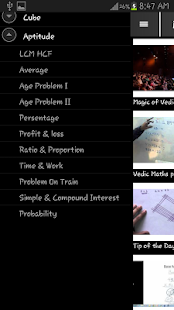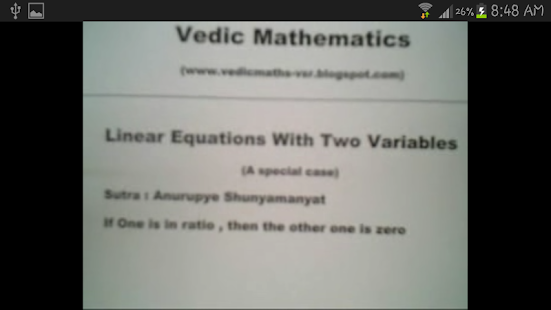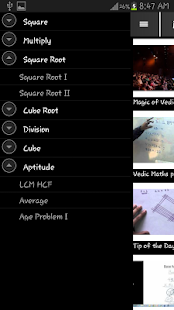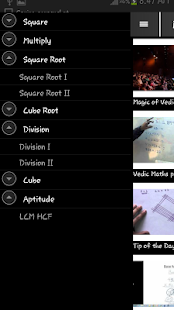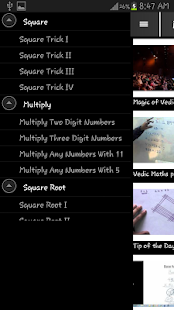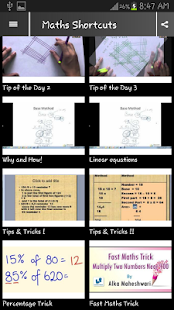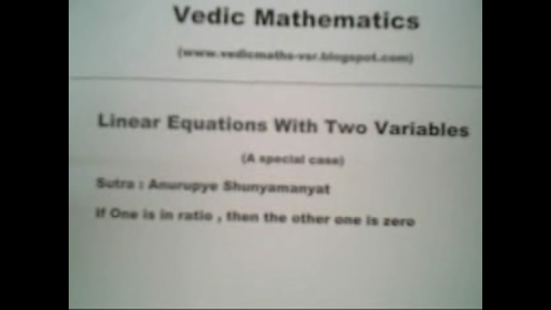Maths Shortcut Tricks (Vedic)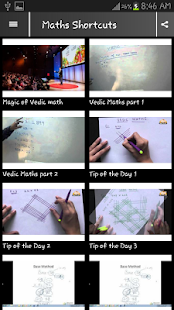This app gives you many videos demonstrating Maths problem solving shortcut tricks .

You can easily find Multiply , square roots , cube roots , Division Tricks and Aptitude chapters Like ( Percentage , ratio and proportion , age , speed distance and time, etc.)